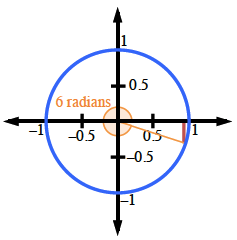Home > A2C > Chapter 8 > Lesson 8.1.6 > Problem8-94

8-94.
1. Draw a picture of an angle that measures 6 radians. Homework Help ✎

1. Approximately how many degrees is this?

2. Using only your picture, estimate the sine of 6 radians.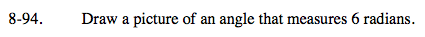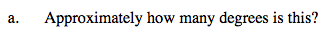Notice that 2π radians ≈ 2(3.14) ≈ 6.27.
So it's a little less than 360°.

$\frac{6}{2 \pi} = \frac{x}{360}$

6 radians ≈ 344°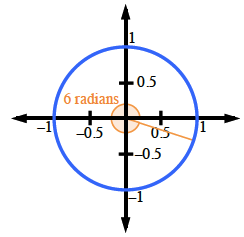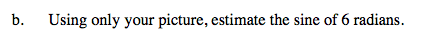sin(6) = −0.3